## Example Questions

### Example Question #1 : How To Find Range

Quantitative Comparison

A set of numbers, Set A, has a mean of 4 and a standard deviation of 2.  Another set, Set B, has a mean of 100 and a standard deviation of 20.

Quantity A: dispersion of numbers in Set A

Quantity B: dispersion of numbers in Set B

Quantity A is greater.

The two quantities are equal.

The relationship cannot be determined from the information given.

Quantity B is greater.

Quantity B is greater.

Explanation:

The standard deviation tells us how much variation, or dispersion, there is on average between the numbers in a set and their mean.  Therefore Quantity B is greater, because Set B has a larger standard deviation than Set A.

### Example Question #2 : How To Find Range

Find the range of the following set of numbers: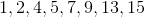Cannot be determined.Explanation:

The range is the difference between the largest and smallest numbers of a set. The biggest number is 15 and the smallest is 1, so our range is 15 – 1 = 14.

### Example Question #3 : How To Find Range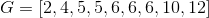Quantity A:

The sum of the median and mode of Set G

Quantity B:

The range of Set G

The two quantities are equal.

The relationship cannot be determined from the information given.

Quantity B is greater.

Quantity A is greater.

Quantity A is greater.

Explanation:

The median is the middle value in a set of numbers, and the mode is the number that occurs.

In Column A, the median is 6 and the mode is 6, so the sum of the median and the mode of Set G is 12.

The range is the difference between the highest and lowest numbers of the set.

In Column B, the range is 12 – 2 = 10.

Column A is greater than Column B.

### Example Question #4 : How To Find Range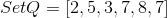Quantity A: The range of Set Q.

Quantity B: The median of Set Q.

Quantity A is greater.

Quantity B is greater.

The two quantities are equal.

The relationship cannot be determined.

The two quantities are equal.

Explanation:

The first step of this problem should be to reorder Set Q into numerical order: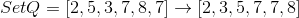The range of a set is the largest value minus the lowest value, so for this set, the range is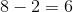The median of a set depends on whether or not there is an even or odd amount of numbers in the set. For a set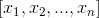withvalues:

Odd: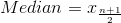Even: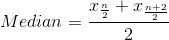Since there is an even number of numbers in this set, the median is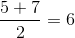The two quantities are equal.

Tired of practice problems?

Try live online GRE prep today.